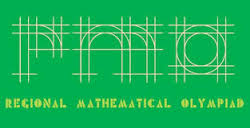# Indian Regional Mathematical Olympiad Problem 1

Probability Level 2Consider all the 7-digit numbers containing each of the digits 1, 2, 3, 4, 5, 6, 7 exactly once and that are not divisible by 5. They are arranged in a list in increasing order. Find the 2000th number in this list.

×